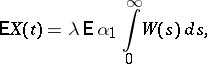# Shot effect

A mathematical description of voltage fluctuations at the output of a linear system at the input of which there are random perturbations produced at random moments of time. If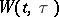is the output of the system at timeresulting from a single pulse applied at time, the shot effect may be described by a stochastic process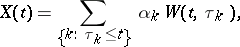where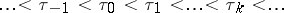are the arrival moments of pulses, while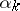are random variables characterizing the magnitudes of the intensities of the pulses. In the particular case when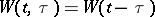,,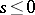, theare independent, uniformly-distributed random variables with finite variance, while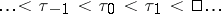forms a Poisson flow of events with parameter, the process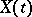is a stationary stochastic process in the narrow sense, with